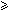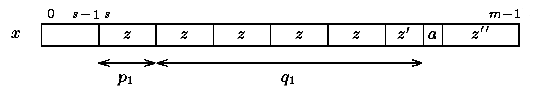Next: Two Way algorithm Up: ESMAJ Prev: Backward Oracle Matching algorithm

# Galil-Seiferas algorithm

Main features
• constant extra space complexity;
• preprocessing phase in O(m) time and constant space complexity;
• searching phase in O(n) time complexity;
• performs 5n text character comparisons in the worst case.
Description

Throughout this section we will use a constant k. Galil and Seiferas suggest that practically this constant could be equal to 4.

Let us define the function reach fori < m as follows: reach(i)=i+max{i’m-i : x[0 .. i’]=x[i+1 .. i’+i+1]}

Then a prefix x[0 .. p] of x is a prefix period if it is basic and reach(p)kp.

The preprocessing phase of the Galil-Seiferas algorithm consists in finding a decomposition uv of x such that v has at most one prefix period and |u|=O(per(v)). Such a decomposition is called a perfect factorization.

Then the searching phase consists in scanning the text y for every occurrences of v and when v occurs to check naively if u occurs just before in y.

In the implementation below the aim of the preprocessing phase (functions newP1, newP2 and parse) is to find a perfect factorization uv of x where u=x[0 .. s-1] and v=x[s .. m-1]. Function newP1 finds the shortest prefix period ofx[s .. m-1]. Function newP2 finds the second shortest prefix period of x[s .. m-1] and function parse increments s.

Before calling function search we have:x[s .. m-1] has at most one prefix period;if x[s .. m-1] does have a prefix period, then its length is p1;x[s .. s+p1+q1-1] has shortest period of length p1;x[s .. s+p1+q1] does not have period of length p1.

The pattern x is of the form x[0 .. s-1]x[s .. m-1] where x[s .. m-1] is of the form zzaz” with z basic, |z|=p1, z’ prefix of z, za not a prefix of z and |zz’| =p1+q1 (see figure 24.1).Figure 24.1: A perfect factorization of x.

It means that when searching for x[s .. m-1] in y:if x[s .. s+p1+q1-1] has been matched a shift of length p1 can be performed and the comparisons are resumed with x[s+q1];otherwise if a mismatch occurs with x[s+q] with qp1+q1 then a shift of length q/k+1 can be performed and the comparisons are resumed with x.

This gives an overall linear number of text character comparisons.

The preprocessing phase of the Galil-Seiferas algorithm is in O(m) time and constant space complexity. The searching phase is in O(n) time complexity. At most 5n text character comparisons can be done during this phase.

The C code

All the variables are global.

```char *x, *y;
int k, m, n, p, p1, p2, q, q1, q2, s;

void search() {
while (p <= n - m) {
while (p + s + q < n && x[s + q] == y[p + s + q])
++q;
if (q == m - s && memcmp(x, y + p, s + 1) == 0)
OUTPUT(p);
if (q == p1 + q1) {
p += p1;
q -= p1;
}
else {
p += (q/k + 1);
q = 0;
}
}
}

void parse() {
while (1) {
while (x[s + q1] == x[s + p1 + q1])
++q1;
while (p1 + q1 >= k*p1) {
s += p1;
q1 -= p1;
}
p1 += (q1/k + 1);
q1 = 0;
if (p1 >= p2)
break;
}
newP1();
}

void newP2() {
while (x[s + q2] == x[s + p2 + q2] && p2 + q2 < k*p2)
++q2;
if (p2 + q2 == k*p2)
parse();
else
if (s + p2 + q2 == m)
search();
else {
if (q2 == p1 + q1) {
p2 += p1;
q2 -= p1;
}
else {
p2 += (q2/k + 1);
q2 = 0;
}
newP2();
}
}

void newP1() {
while (x[s + q1] == x[s + p1 + q1])
++q1;
if (p1 + q1 >= k*p1) {
p2 = q1;
q2 = 0;
newP2();
}
else {
if (s + p1 + q1 == m)
search();
else {
p1 += (q1/k + 1);
q1 = 0;
newP1();
}
}
}

void GS(char *argX, int argM, char *argY, int argN) {
x = argX;
m = argM;
y = argY;
n = argN;
k = 4;
p = q = s = q1 = p2 = q2 = 0;
p1 = 1;
newP1();
}

```
The example

Preprocessing phase

p=0,  q=0,  s=0,  p1=7,  q1=1.

Searching phase

References
• CROCHEMORE, M., RYTTER, W., 1994, Text Algorithms, Oxford University Press.
• GALIL Z., SEIFERAS J., 1983, Time-space optimal string matching, Journal of Computer and System Science 26(3):280-294.Next: Two Way algorithm Up: ESMAJ Prev: Backward Oracle Matching algorithm

e-mails: {Christian.Charras, Thierry.Lecroq }@laposte.net
Tue Jan 14 15:03:31 MET 1997